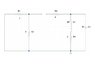# Finding the Equivalent Resistance of a Circuit

• wiz0r
In summary, the homework statement is asking for help to find the equivalent resistance between the connectors at the top of the circuit.f

## Homework Statement

I need to find the equivalent resistance of the following circuit that can be found in the attachment. Also, I need to find if the C, L and Req are in series or parallel.

## Homework Equations

Rs = R1 + R2 + R3 + ... + Rn

Rp = (R1 * R2 * ... * Rn) / (R1 + R2 + ... + Rn)

## The Attempt at a Solution

Okay, well, since there's no current passing through R1, R2, and R3, I assume that Req = R4. Therefore, the capacitance, inductance and the equivalent resistance are in Series.

Am I right? Or is there something wrong?

Any help will be gladly appreciated!

#### Attachments

•resis_equiv.JPG
17.7 KB · Views: 515
Aren't you supposed to find the equivalent resistance between the two connectors at the top?
Having one point of the circuit grounded doesn't really make sense.
is this a DC or an AC problem, where you have to find the equivalent impedance?

hello, kamerling.

the problem I'm trying to solve is a little more difficult than what it seems.

it is a AC problem, more specific it is a second order transient RLC circuit.

the original circuit can be found in the attachment of this post.

the first step to solve the problem is finding if R, L and C are either in Series or Paralel. That's what I'm doing.

I remove the voltage source by a short and the current source by an open circuit, then assume the switch will be opened at t>0, so there will be an open circuit where the switch was.

after doing that, i got the circuit that i posted on my first post. so, I'm trying to find if the Req, L, and C, are in series or parallel to be able to completely solve the problem.

EDIT: The ground isn't supposed to be there, I apologize. I edited the original circuit and left it there, sorry!

#### Attachments

Last edited:
I don't think that calculating an equivalent resistance is going to be much help. You can replace R3, R4, L1 and C1 with an equivalent complex impedance. L1 and R4 are in series, the resulting impedance is parallel with C1 and the result from that is in series with R3. you should be able to see that before you get to AC problems.
I rather wonder what the question is
A transient AC problem would normally involve kirchhofs loop rule and differential equations, using V = L(dI/dt) for the inductor and I = C(dV/dt) for the capacitor